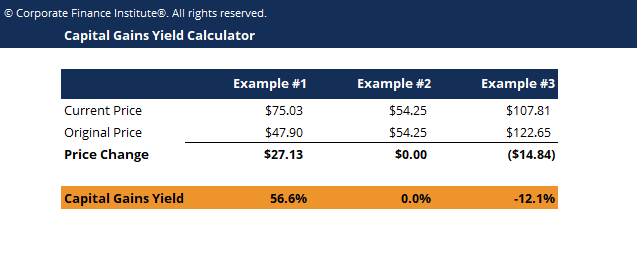# Capital Gains Yield Calculator

## Capital Gains Yield Calculator

This capital gains yield calculator demonstrates how to calculate the capital gains yield using the purchase price and current price of a stock.

Below is a screenshot of the capital gains yield calculator:### Capital Gains Yield Calculator

Capital gains yield (CGY) is the price appreciation on an investment or a security expressed as a percentage.

The formula for calculating capital gains yield is:  CGY = (current price – original price) / original price x 100

Capital Gain is the component of total return on an investment, which occurs as a result of a rise in the market price of the security.

Because the calculation of Capital Gain Yield only involves the market price of a security over time, it can be used to analyze the degree of fluctuation in the market price of a security.

### More Free Templates

For more resources, check out our business templates library to download numerous free Excel modeling, PowerPoint presentation and Word document templates.

• Excel Modeling Templates
• PowerPoint Presentation Templates
• Transaction Document Templates

### Financial Analyst Certification

Become a certified Financial Modeling and Valuation Analyst (FMVA)® by completing CFI’s online financial modeling classes and training program!